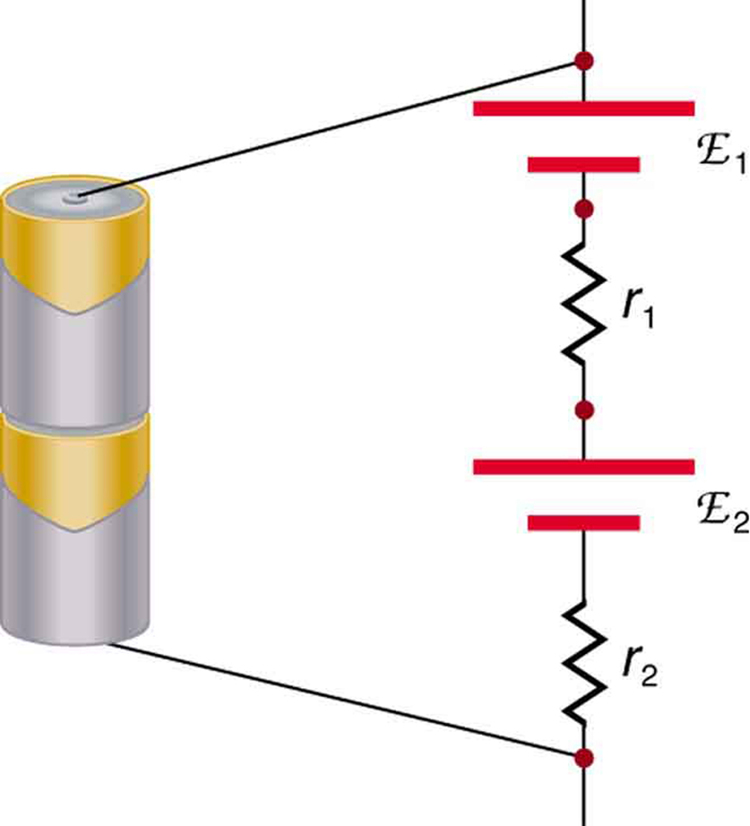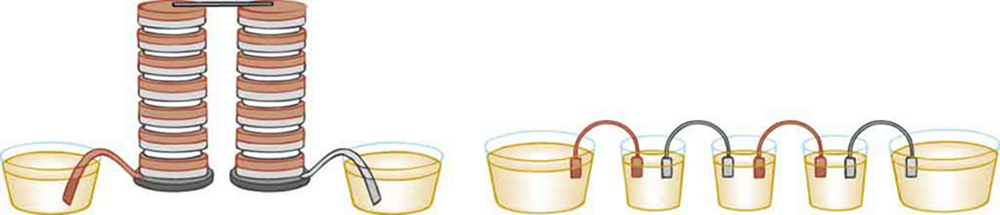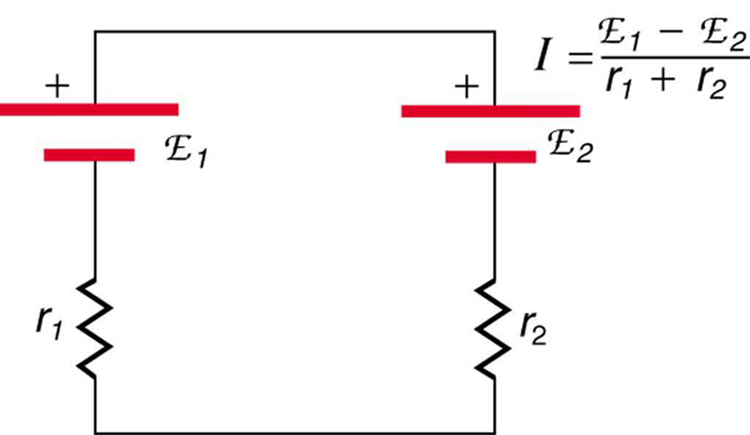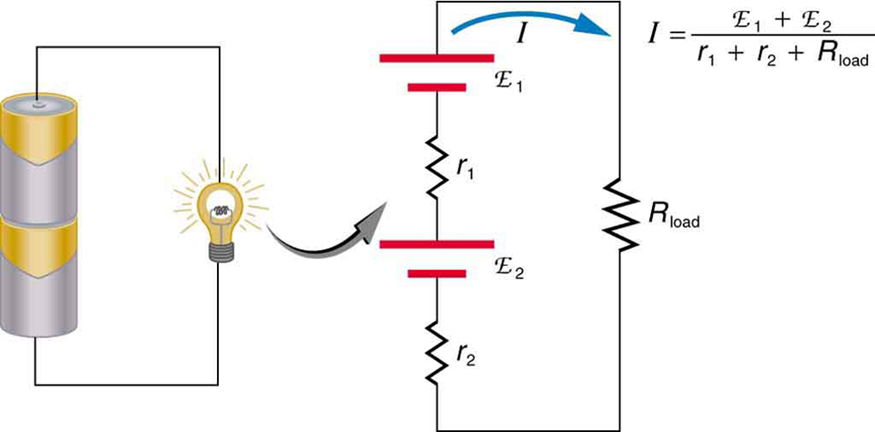# 21.2 Electromotive force: terminal voltage  (Page 5/12)

 Page 5 / 12

But if the cells oppose one another, such as when one is put into an appliance backward, the total emf is less, since it is the algebraic sum of the individual emfs.

A battery is a multiple connection of voltaic cells, as shown in [link] . The disadvantage of series connections of cells is that their internal resistances add. One of the authors once owned a 1957 MGA that had two 6-V batteries in series, rather than a single 12-V battery. This arrangement produced a large internal resistance that caused him many problems in starting the engine.A series connection of two voltage sources. The emfs (each labeled with a script E) and internal resistances add, giving a total emf of emf 1 + emf 2 size 12{"emf" rSub { size 8{1} } +"emf" rSub { size 8{2} } } {} and a total internal resistance of r 1 + r 2 size 12{r rSub { size 8{1} } +r rSub { size 8{2} } } {} .Batteries are multiple connections of individual cells, as shown in this modern rendition of an old print. Single cells, such as AA or C cells, are commonly called batteries, although this is technically incorrect.

If the series connection of two voltage sources is made into a complete circuit with the emfs in opposition, then a current of magnitude $I=\frac{\left({\text{emf}}_{1}–{\text{emf}}_{2}\right)}{{r}_{1}+\phantom{\rule{0.25em}{0ex}}{r}_{2}}$ flows. See [link] , for example, which shows a circuit exactly analogous to the battery charger discussed above. If two voltage sources in series with emfs in the same sense are connected to a load ${R}_{\text{load}}$ , as in [link] , then $I=\frac{\left({\text{emf}}_{1}+\phantom{\rule{0.25em}{0ex}}{\text{emf}}_{2}\right)}{{r}_{1}+\phantom{\rule{0.25em}{0ex}}{r}_{2}+{R}_{\text{load}}}$ flows.These two voltage sources are connected in series with their emfs in opposition. Current flows in the direction of the greater emf and is limited to I = emf 1 − emf 2 r 1 + r 2 size 12{I= { { left ("emf" rSub { size 8{1} } - "emf" rSub { size 8{2} } right )} over {r rSub { size 8{1} } +r rSub { size 8{2} } } } } {} by the sum of the internal resistances. (Note that each emf is represented by script E in the figure.) A battery charger connected to a battery is an example of such a connection. The charger must have a larger emf than the battery to reverse current through it.This schematic represents a flashlight with two cells (voltage sources) and a single bulb (load resistance) in series. The current that flows is I = emf 1 + emf 2 r 1 + r 2 + R load size 12{I= { { left ("emf" rSub { size 8{1} } - "emf" rSub { size 8{2} } right )} over {r rSub { size 8{1} } +r rSub { size 8{2} } +R rSub { size 8{"load"} } } } } {} . (Note that each emf is represented by script E in the figure.)

## Take-home experiment: flashlight batteries

Find a flashlight that uses several batteries and find new and old batteries. Based on the discussions in this module, predict the brightness of the flashlight when different combinations of batteries are used. Do your predictions match what you observe? Now place new batteries in the flashlight and leave the flashlight switched on for several hours. Is the flashlight still quite bright? Do the same with the old batteries. Is the flashlight as bright when left on for the same length of time with old and new batteries? What does this say for the case when you are limited in the number of available new batteries?

[link] shows two voltage sources with identical emfs in parallel and connected to a load resistance. In this simple case, the total emf is the same as the individual emfs. But the total internal resistance is reduced, since the internal resistances are in parallel. The parallel connection thus can produce a larger current.

An American traveler in New Zealand carries a transformer to convert New Zealand’s standard 240 V to 120 V so that she can use some small appliances on her trip.
What is the ratio of turns in the primary and secondary coils of her transformer?
nkombo
How electric lines and equipotential surface are mutually perpendicular?
The potential difference between any two points on the surface is zero that implies È.Ŕ=0, Where R is the distance between two different points &E= Electric field intensity. From which we have cos þ =0, where þ is the angle between the directions of field and distance line, as E andR are zero. Thus
sorry..E and R are non zero...
By how much leeway (both percentage and mass) would you have in the selection of the mass of the object in the previous problem if you did not wish the new period to be greater than 2.01 s or less than 1.99 s?
what Is linear momentum
why no diagrams
where
Fayyaz
Myanmar
Pyae
hi
Iroko
hello
Abdu
Describe an experiment to determine short half life
what is science
it's a natural phenomena
Hassan
sap
Emmanuel
please can someone help me with explanations of wave
Benedine
A 20MH coil has a resistance of 50 ohms and us connected in series with a capacitor to a 520MV supply
what is physics
it is the science which we used in our daily life
Sujitha
Physics is the branch of science that deals with the study of matter and the interactions it undergoes with energy
Junior
it is branch of science which deals with study of happening in the human life
AMIT
A 20MH coil has a resistance of 50 ohms and is connected in series with a capacitor to a 250MV supply if the circuit is to resonate at 100KHZ, Determine 1: the capacitance of the capacitor 2: the working voltage of the circuit, given that pie =3.142
Musa
Physics is the branch of science that deals with the study of matter and the interactions it undergoes with energy
Kelly
Heat is transfered by thermal contact but if it is transfered by conduction or radiation, is it possible to reach in thermal equilibrium?
Yes, It is possible by conduction if Surface is Adiabatic
Astronomy
Yeah true ilwith d help of Adiabatic
Kelly
what are the fundamentals qualities
what is physic3
Kalilu
what is physic
Kalilu
Physics? Is a branch of science dealing with matter in relation to energy.
Moses
Physic... Is a purging medicine, which stimulates evacuation of the bowels.
Moses
are you asking for qualities or quantities?
Noman
give examples of three dimensional frame of reference
Universe
Noman
Yes the Universe itself
Astronomy
Examine different types of shoes, including sports shoes and thongs. In terms of physics, why are the bottom surfaces designed as they are? What differences will dry and wet conditions make for these surfaces?
sports shoes are designed in such a way they are gripped well with your feet and their bases have and high friction surfaces, Thong shoes are for comfort, these are easily removed and light weight. these are usually low friction surfaces but in wet conditions they offer greater friction.
Noman
thong sleepers are usually used in restrooms.
Noman
what is wave
The phenomenon of transfer of energy
Noman
how does time flow in one dimension
yeah that was a mistake
Lord
if it flows in three dimensions does it mean if an object theoretically moves beyond the speed of light it won't experience time
Lord
but if an object moves beyond the speed of light time stops right for it
Lord
yes but at light speed it ceases
Lord
yes it always flow from past to future.
NomanByByByBy OpenStaxBy OpenStaxBy Stephen VoronBy Sarah WarrenBy Mary CohenBy OpenStaxBy OpenStaxBy Jonathan LongBy Madison Christian